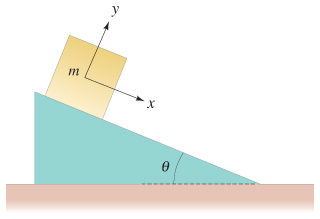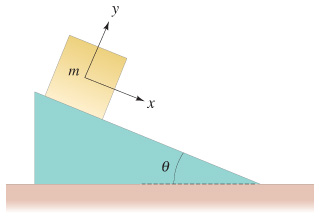# Problem: The block shown in the figure has mass m = 7.5 kg and lies on a fixed smooth frictionless plane tilted at an angle θ = 23.0° to the horizontal.(a) Determine the acceleration of the block as it slides down the plane.(b) If the block starts from rest 13.0 m up the plane from its base, what will be the block's speed when it reaches the bottom of the incline?

###### FREE Expert Solution

This problem requires us to determine the acceleration and final velocity after some displacement in an inclined plane.

Remember that for objects on inclined planes, we use a coordinate system where the +x-axis points parallel to the ramp. The object accelerates due to a component of gravity along the ramp:

where θ is the angle between the plane's surface and the horizontal. This acceleration is positive, since the component of gravity is acting along the positive x-axis.

Since the acceleration is constant, we can apply the four kinematics equations. As always for kinematics problems, we'll follow these steps:

1. Identify the target variable, knowns, and Unknowns for each part of the problem—remember that only 3 of the 5 variablesy, v0, vf, a, and t) are needed to solve any kinematics problem, and we already know what a is.
2. Choose a UAM equation with only one unknown, which should be our target variable.
3. Solve the equation for the target variable, then substitute known values and calculate the answer.

The four UAM equations are:

(a) First, the problem asks for the acceleration of the block. We'll use the equation a = g sinθ. Assuming g = 9.81 m/s2:

100% (415 ratings)###### Problem Details

The block shown in the figure has mass m = 7.5 kg and lies on a fixed smooth frictionless plane tilted at an angle θ = 23.0° to the horizontal.
(a) Determine the acceleration of the block as it slides down the plane.
(b) If the block starts from rest 13.0 m up the plane from its base, what will be the block's speed when it reaches the bottom of the incline?